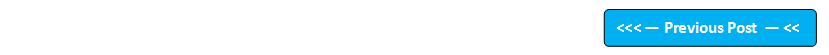## Blog Pages

### 247. Double Wrapper ClassPre-requisites -

Double wrapper class is used to wrap the double primitive type into an object, whenever we need primitive type to be used as an object.

Example of wrapping double primitive type into an object using Double wrapper class -

double pi = 3.14159;
Double p1 = new Double(pi);  //Wrapping the double primitive type variable pi  into Double wrapper class type object p1

Example of unwrapping the Double wrapper class type object pi into double primitive type variable p -

double p = p1.doubleValue( ) //Unwrapping the Double wrapper class type object p1 using doubleValue( ) method to double primitive data type variable p

Lets implement this on Eclipse IDE -

1. Launch Eclipse IDE, create a new Java Class 'DoubleWrapperClassDemo.java' with main( ) method in the existing Java Project 'Wrapper Classes' as shown below -

2. Declare a double primitive class type variable pi and assign it a value as shown below -

3. Now wrap the double primitive data type variable pi into a Double wrapper class type object p1 as shown below -

4. Now print the wrapped Double class type object 'p1' as shown below -

5. Save and Run the Java Class 'DoubleWrapperClassDemo.java' and observe that the double primitive data type value 3.14159 is printed using the wrapped object 'p1' in the output as shown below -

6. Now unwrap the Double class object p1 back to double primitive data type as shown below -

7. Print the unwrapped double primitive data type 'p' as shown below -

8. Save & Run the Java Class 'DoubleWrapperClassDemo.java' and observe that the double primitive data type value 3.14159 is printed using the unwrapped double primitive data type 'p' in the output as shown below -

Hence we can wrap the double primitive data type into an object using Double Wrapper Class and unwrap the object back to the double primitive data type using the doubleValue( ) method.